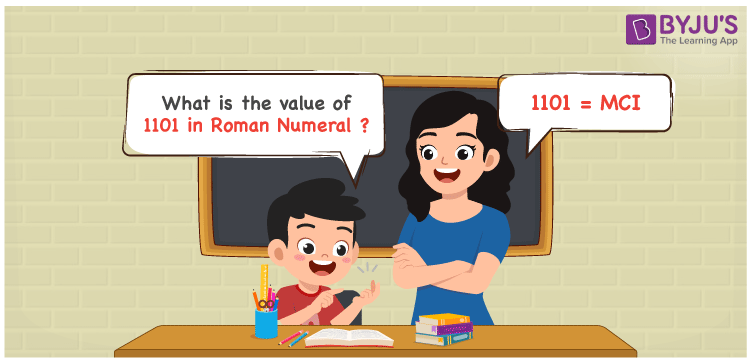# 1101 in Roman Numerals

1101 in Roman Numerals is MCI. Any Roman numeral uses only the 7 primary symbols which are I, V, X, L, C, D, and M, Where M represents 1000 and D represents 500 . What are Roman numerals? can be referred for more details. The details on how to write 1101 is given in the next section of this article.

 Number Roman Numeral 1101 MCI

## How to Write 1101 in Roman Numerals?To convert 1101 in Roman Numerals, we need to represent 1101 as the sum of the fundamental symbols. 1101 can be broken down as the sum of 1000 and 100. Since M is the symbol for 1000, and C is the symbol for 500 and I for 1, 1101 will be MCI. The addition rule of Roman numerals is followed here.

1101 = 1000 + 100 + 1

1101 = M + C + I

1101 = MCI

## Video Lesson on Roman Numerals## Frequently Asked Questions on 1101 in Roman Numerals

Q1

### How to write the number 1101 in Roman Numerals?

1101 in Roman Numerals is written as MCI.
Q2

### Is MCI the same as CM in Roman Numerals?

No. MCI equals to value 1101 and CM equals to the value 900
Q3

### What is the year 1101 in Roman numerals?

The year 1101 in Roman Numerals is MCI.